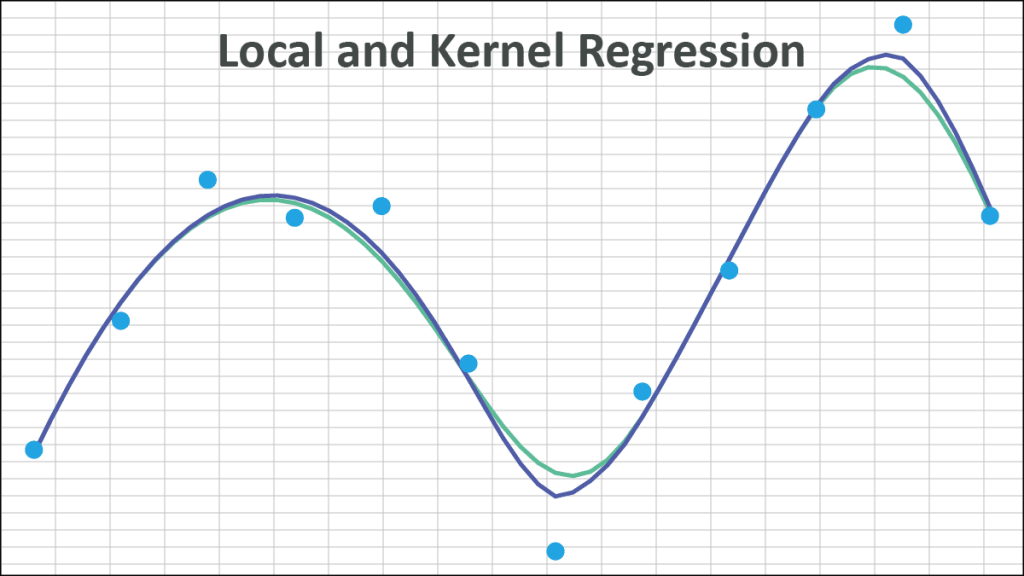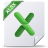# Local & Kernel Regression (Non-Parametric)

This issue was inspired by an inquiry from a customer regarding two of our non-parametric data fitting functions: NxLOCREG(.) and NxKREG(.) which are used for Local Regression and Kernel Regression respectively. The customer wrote:

Inquiry 1:

The NxLOCREG(.) function implements a localized regression algorithm – LOESS (or LOWESS), aka the Savitzky–Golay filter.

The NxKREG(.) function implements a weighted least squares regression.

The two functions are very similar, but NxLOCREG(.) has an additional argument: Alpha or H, which can take a value between zero (exclusive) and 1 (inclusive). As a result, the NxLOCREG function selects the nearest H*N data points, while NxKREG (.) always includes the whole data set (i.e., N).

In more detail, given a target value X, the kernel regression procedure goes as follows:

• Calculate the distance between the target value and the data points in the sample.
• Sort data points based on their distance in ascending order.
• Select the regression input data set:
• (NxLOCREG case) Select the nearest (i.e., first) H*N data points.
• (NxKREG case) Select the whole N data points.
• Calculate the weights of the selected data points using the given Kernel function.
• Conduct the weighted least squares regression model.
• Calculate the regression value at value X.

The procedure/algorithm uses the H*N data points that are closest to the target value, so every value will construct its own regression model with a (possibly) different data set.

If Alpha = 1.0, keeping all other arguments the same, the NxKREG(.) and NxLOCREG(.) functions will return identical results.

Currently, the NxLOCREG and NxKREG(.) functions support polynomials of order up to 3 (cubic).

Regardless of the chosen weighting kernel function, we need a value for the bandwidth (aka the smoothing parameter). NumXL uses a data-based bandwidth selection method, minimizing the RMSE for cross-validation.

In a nutshell, for a given bandwidth, we calculate the regression value for each data point (using leave-one out cross-validation) and compute the RMSE between regression values and the actual values.

Inquiry 2:

This will depend on how you define “Spike.” If Spike is a reference to an outlier (a large Y value), then this method will include data points with outliers. Still, these data points may be weighted down based on their Euclidean distance from the target value.

On the other hand, if Spike is a reference to a remote data point along the x-domain, then those remote data points will be weighted down significantly in the regression.

Inquiry 3:

Yes, we believe that Microsoft Excel uses a LOESS algorithm to generate the smoothed lines in the scatterplot. You can use the NxLOCREG(.) function, but we can’t be sure of the parameter values used, e.g., the kernel function, polynomial order, or the alpha value.

Download a fully functional, free 14-day trial of NumXL to test or apply these functions to your own data.
Make sure to look into the Tips & Tricks and Data Fitting sections for more articles that might interest you.

## Files Examples